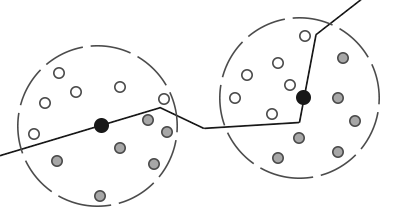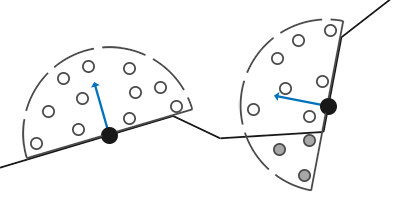# 基于屏幕空间的实时全局光照（Real-time Global Illumination Based On Screen Space）

$E_{p}(x) = \Phi_p \frac{\max (\mathbf{n_p} \cdot normalize(x-x_p),0)\max (\mathbf{n} \cdot normalize(x_{p}-x),0)}{\left\|x-x_{p}\right\|^{2}}$$$\Phi$$ 是次级光源 patch 的 radiant flux，$$\mathbf{n_p}$$$$x_p$$ 的法线 ，$$\mathbf{n}$$$$x$$ 的法线

$E(x) =\sum \ E_{p}(x)$1. 阴影生成 pass：在光源摄像机渲染 shadow map （往往只记录了深度）的时候，顺便额外记录 世界坐标 $$x_p$$ 、法线 $$n_p$$、 接受的直接光源 radiant flux $$\Phi_p$$。那么就可以认为 shadow map 的一个 texel 对应一块patch ，从而这张 shadow map 就包含了所有次级光照 patch 的信息了 。

$\Phi_p=\Phi(u_p,v_{p})= I * c_p$

$$u_p、v_p$$$$x_p$$ 在 shadow map 上的纹理坐标。1. 主渲染pass：在 pixel shader 阶段，计算出 $$x$$ 对应的 shadow map uv坐标，并取该坐标周围若干个 texel （这些正是我们要采样的次级光源点）对应的 世界坐标 $$x_p$$ 、法线 $$n_p$$、 接受的直接光源radiant flux $$\Phi_p$$ ，它们将对 $$x$$ 的渲染造成间接光照影响：

$L_{indirect}(x,\mathbf{v}) = \frac{E(x)}{\pi} = \frac{{\sum_{\text {texels p}} \ E_{p}(x)}}{\pi}$RSM效果图：## RSM 重要性采样

RSM 假定，离 shading point x 近的点更可能给 x 的光照贡献大，而远的点给 x 的光照贡献小。$(u,v)=\left(s+r_{\max } \xi_{1} \sin \left(2 \pi \xi_{2}\right), t+r_{\max } \xi_{1} \cos \left(2 \pi \xi_{2}\right)\right)$

$importance = (\xi_{1})^2$

## RSM 的应用与缺陷• 仅支持 one-bounce 间接光照效果
• RSM 假设次级光源面均是 diffuse 的，这会影响图像的正确性（当然大部分情况下还是可以接受的）

• 作为廉价的GI方法，常被用于做单个重要光源的GI效果（例如手电筒）# Screen Space Ambient Occulsion（SSAO）AO 的基本公式：

$A(x) = 1-\frac{1}{\pi}\int{}V(x,w_i) cos\theta_i \mathrm{d}w_i$

AO 往往只代表一个简单的光线入射遮挡比例，乘于它意味着需要把 shading point 当作 diffuse 的表面来看待（即与观察方向无关，出射到哪都是 $$\frac{1}{\pi}$$ 的 irradiance）。1. 在该点一定半径内的球型范围内随机采样 N 个点，然后这些采样点将与 depth buffer 对应的深度作比较：若采样点的深度小于 depth buffer 对应位置的深度，则说明该采样点被遮蔽了

2. 根据所有被遮蔽得到采样点数量 $$Occ$$，计算出 AO 为 $$A(p) = \frac{Occ}{N}$$

$L_{indirect}(x) = \frac{1-A(x)}{\pi} \int_{\Omega^{+}} L_{\mathrm{environment}}\left(\mathrm{x}, \omega_{i}\right) {\rho} \cos \theta_{i} \mathrm{~d} \omega_{i}$

$$\rho$$ 为 albedo。

SSAO 效果图（左为关闭SSAO效果，右为开启SSAO效果，可以看到物体交界处等地方多了更多的暗部细节）：## SSAO Blur## SSAO 半球采样

vec3 rand;				// 在球形上的随机坐标
rand = sign(dot(n,rand))*rand;	// 在半球上的随机坐标## SSAO 的应用与缺陷

• 仅包含屏幕表面的几何信息不能表示完全正确的 visibility，因此 AO 效果不那么准确（相对于预计算AO贴图）• 仅支持短距离的物体遮蔽效果

• 廉价的GI效果，提升画面的暗部细节，大部分游戏都会将其纳入一种画面增强选项，虽然之后有性能和效果更好的 HBAO 算法作为取代。

# Screen Space Directional Occlusion（SSDO）

Screen Space Directional Occlusion（SSDO） 也是一类与 SSAO 极其相似的屏幕空间 GI 方法，区别在于它们看待光线遮蔽的角度是相反的：

• AO 认为 shading point 朝外的光线打到物体几何表面时，相当于外部环境光被这个表面遮挡了，因此（对于下面这幅图） AO 将红色部分视为间接光照来源，黄色部分视为损失的间接光照
• 而 DO 认为 shading point 朝外的光线打到物体几何表面时，相当于受到了表面的间接光照，因此（对于下面这幅图） DO 会将黄色部分视为间接光照来源，红色部分视为损失的间接光照SSDO 需要依赖屏幕的 color, depth 信息来完成。

SSDO 算法流程的思路也和 SSAO 相似：对于某个 shading point，1. 先在 shading point 一定半径内的半球型范围内随机采样 N 个点，然后这些采样点将与 depth buffer 对应的深度作比较：若采样点的深度小于 depth buffer 对应位置的深度，则说明该采样点被遮蔽了
2. 对于每个被遮蔽的采样点，将该采样点对应的 pixel 视为次级光源 patch，对 shading point 造成间接光照的贡献：

SSDO 计算该 GI 的时候会把物体几何表面所有 pixels（也包括 shading point 本身）都将假设为 diffuse 表面

$E_p(x) = L_{direct}(p)(1-V_p(x)) \frac{{Area}(p)\cos\theta_{s_i}\cos\theta_{r_i}}{d^2_i}$

$$Area(p)$$ 即为 pixel p 的片元面积，可以通过 p 的深度算出（p越远，对应的片元面积越大）

$E(x) = \sum_{i=0}^N E_{p_i}(x)$

$L_{indirect}(x) = \frac{\rho}{\pi} E(x)$

SSDO 效果图：## SSDO 的应用与缺陷

• 仅包含屏幕表面的几何信息仍然不能表示完全正确的 visibility，即会缺失屏幕看不到的平面信息（对于有颜色的GI效果很容易看出artifact）• 仅支持短距离GI效果，而无法展示长距离的GI• 仅支持 one-bounce 间接光照

# Horizon Based Ambient Occlusion（HBAO）

HBAO 就是基于方向角覆盖率的思想出发：在 shading point 上往各个方向进行 ray tracing（为了开销低，实际用的是ray marching），找到与 shading point 切平面夹角最大且 hit success（意味着遇到遮挡物）的 ray 方向，通过该 ray 方向和切平面的夹角 $$\alpha$$ 就可以得到遮蔽率 ：

$A = \int^{\alpha}_{\phi=0}\cos(\phi)\mathrm{d}\phi = \sin(\alpha)$

$A \approx \int^{h}_{\phi=t}\cos(\phi)\mathrm{d}\phi = \sin(h)-\sin(t)$HBAO 的具体算法流程为：

1. 对于 shading point p，我们先屏幕空间上采样四个方向，它们对应 $$\theta_1$$,$$\theta_2$$,$$\theta_3$$,$$\theta_4$$
• 初始的四个方向都是轴对齐的十字（虚线），然后对整个十字进行一个随机旋转角度，就可以得到当前帧的四个采样方向（实线）1. 对于某个采样方向 $$\theta$$

• shading point 上沿 $$\theta$$ 方向进行 ray marching，最终找到可遇到遮蔽物与 view 平面最高仰角的 sin 值：

$tan(h(\theta)) = \frac{RayDir.z}{\sqrt{(RayDir.x)^2+(RayDir.y)^2}}$

$\sin(h(\theta)) = \frac{\tan(h(\theta))}{\sqrt{1+\tan^2(h(\theta))}}$

如下图例子，找到的最高仰角为 $$S_3$$ 方向上的。• 也顺便根据 ray tracing 结果对应的 hit dist $$r(\theta)$$，计算出本次 $$\theta$$ 角切面的样本权重为：

$W(\theta) = max(0,1-\frac{r(\theta)}{R_{max}})$

• 根据 shading point 的 normal 算出其切线 tangent，算出切线与 view 平面的夹角的 sin 值，同理可算出 $$sin(t(\theta))$$
1. 最终计算 AO 为：

\begin{aligned} A(x) & = \frac{1}{2\pi} \int_{\theta = -\pi}^{\pi} (\int^{h(\theta)}_{\phi=t(\theta)} \cos(\phi) \mathrm{d}\phi)W(\theta) \mathrm{d}\theta \\& = \frac{1}{2\pi} \int_{\theta = -\pi}^{\pi} ( \sin(h(\theta))-\sin(t(\theta)))W(\theta) \mathrm{d}\theta \end{aligned}

1. 最后对 AO 图像进行一个空间滤波（或者说模糊）来降低噪声## HBAO 的 Normal 问题

shading point 在利用 normal 计算切线 tangent 的时候，最好使用 face normal（即三角面法线）而非 interpolated normal（通过顶点插值得到的法线）：如果使用 interpolated normal ，那么在模型凹角处容易出现 artifacts。## HBAO 的采样

HBAO 在选择屏幕空间采样方向和在 ray marching 的时候可以使用开销极其低廉的预定义样本（可通过数组定义）：每帧随机选择一种十字采样方向（共有5种），选定后每个采样方向 ray marching 也只步进两次。## HBAO 的不连续问题$W(\theta) = max(0,1-\frac{r(\theta)}{R_{max}})$

$W(\theta) = max(0,1-(\frac{r(\theta)}{R_{max}})^2)$## HBAO 的应用与缺陷

• HBAO 比 SSAO 开销往往更低：由于只需要更低于 SSAO 的样本数，甚至还能达到质量更高的 AO 效果。

• 对于有中空的 AO 情况，HBAO 会把 hit success 的最高仰角以下的方向都认为是被遮挡的，这是与 Ground Truth AO 不符（SSAO 反而更能适应这种中空情形）。# Screen Space Reflection（SSR）/Screen Space Ray Tracing（SSRT）

Screen Space Reflection（SSR）,一类与 ray tracing 思路非常相似的屏幕空间GI方法，因此也有被叫为 Screen Space Ray Tracing（SSRT）SSR 需要用到的屏幕信息：color、normal、depth

SSR 的算法流程：

1. 在第一个 pass 只渲染整个场景的直接光照，得到包含直接光照结果的 color buffer 、normal buffer、 depth buffer。

2. 在第二个 pass 对整个屏幕渲染，对于某个 shading point ，在该点往半球随机方向投射若干条射线（使用 ray marching算法），然后将与射线相交的点 $$\mathrm{p'}$$ 将对 shading point 的间接光照做出贡献（这与渲染方程是一致的）：

$L_{\mathrm{indirect}}\left(\mathrm{p}, \omega_{o}\right) = \int_{\Omega^{+},V=1} L_{}\left(\mathrm{p'}, \omega_{i}\right) f_{r}\left(\mathrm{p}, \omega_{i}, \omega_{o}\right) \cos \theta_{i} \mathrm{~d} \omega_{i}$

其中当射线命中时， $$V = 1$$ ；否则，$$V = 0$$

为了减少计算，这里仍然假设次级光源点是 diffuse 的，这样式子实际可以写成：

$L_{\mathrm{indirect}}\left(\mathrm{p}, \omega_{o}\right) = \int_{\Omega^{+},V=1} \frac{E(\mathrm{p'})}{\pi} f_{r}\left(\mathrm{p}, \omega_{i}, \omega_{o}\right) \cos \theta_{i} \mathrm{~d} \omega_{i}$SSR 效果图：## SSR的 Ray Marching

1. 每次往射线方向走一个步长得到一个测试点，将该测试点变换成屏幕坐标 $$(u,v,z)$$
2. 根据uv坐标取 depth buffer 对应的深度 $$d$$$$z$$ 比较：若 $$z>d$$ ，则说明射线碰到该uv位置上像素点的“柱条”，返还该测试点；否则，重复上述步骤bool RayMarch(vec3 ori, vec3 dir, out vec3 hitPos) {
float step = 1.0;
vec3 lastPoint = ori;
for(int i=0;i<10;++i){
// 往射线方向走一步得到测试点深度
vec3 testPoint = lastPoint + step * dir;
float testDepth = GetDepth(testPoint);
// 测试点的uv位置对应在depth buffer的深度
vec2 testScreenUV = GetScreenCoordinate(testPoint);
float bufferDepth = GetGBufferDepth(testScreenUV);
// 若测试点深度 > depth buffer深度，则说明光线相交于该测试点位置所在的像素柱条
if(testDepth-bufferDepth > -1e-6){
hitPos = testPoint;
return true;
}
// 继续下一次 March
lastPoint = testPoint;
}
return false;
}


## Depth Mipmap 加速 Ray Marching

mip = 0;
while(level>-1)
step through current cell;
if(above Z plane) ++level;
if(below Z plane) --level;## BRDF 重要性采样## 射线结果重用## 预过滤采样结果## SSR/SSRT 的应用与缺陷

• screen space 方法仍然缺失了屏幕所看不到的几何信息• diffuse 情况下，由于要往半球范围均匀采样（不能像specular/glossy那样用importance sampling极大优化采样），容易造成nosiy结果，这时候可能需要牺牲更多的性能来采样更多

• SSR 的渲染效果非常好（前面的方案看起来总像是增强部分的图像效果）
• 通过不同的 brdf 函数，可以自由调成各种反射效果（specular/glossy/diffuse）

# 参考

posted @ 2021-10-31 21:12  KillerAery  阅读(1532)  评论(0编辑  收藏  举报